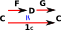# Maths - Category Theory - Weak Equivilance

Weak equivilance provides a simpler way to determine equivilance.

 On the equivilance page we discussed how to determine equivilance by finding two functors. F and G, which give the identity when composed in both directions.Weak equivilance gives us a way to do this using only a functor in one direction F. However this only tells us that an equivilance exists, not the functors which give the equivilance.Given this functor F : C -> D there is an equivalence if and only if the following are all true:

• F is full, i.e. for any two objects c1 and c2 of C, the map HomC(c1,c2) -> HomD(Fc1,Fc2) induced by F is surjective;
• F is faithful, i.e. for any two objects c1 and c2 of C, the map HomC(c1,c2) -> HomD(Fc1,Fc2) induced by F is injective; and
• F is essentially surjective (dense), i.e. each object d in D is isomorphic to an object of the form Fc, for c in C.

## Further Information

Weak equivalence was first used in algebraic topology, in particular, in model category theory as described on the page here.